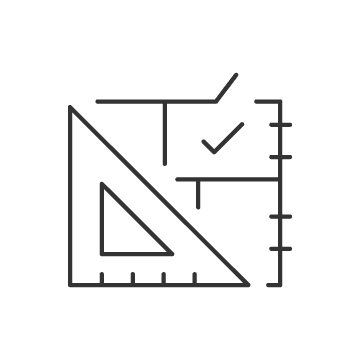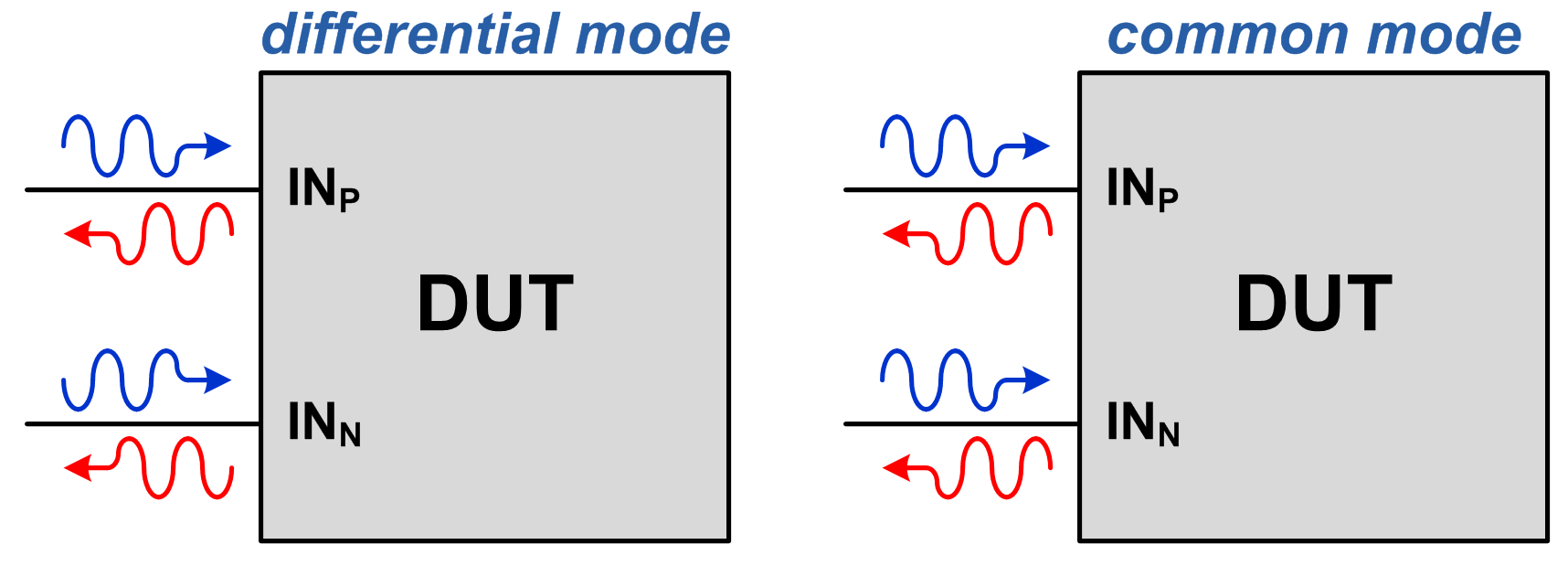# So, what are S-parameters anyway?

Modern high-speed analog-to-digital converters (ADCs) have enabled the direct sampling of radio frequency (RF) signals, which in many cases eliminates the need for mixing stages while increasing system flexibility and capability.

Traditionally, ADC signal and clock inputs have been represented by lumped element models. But for RF sampling converters, this approach is inadequate, since operating frequencies have increased to the point where a distributed representation is required.

In this three-part series, I’ll explain how to use scattering parameters, also known as S-parameters, in the design of direct RF sampling architectures.

Defining S-parameters

S-parameters provide a framework for describing networks based on the ratio of incident and reflected microwaves. This is useful for circuit design, because you can use these ratios to calculate properties such as input impedance, frequency response and isolation. It’s not necessary to know the details of the network, since it’s possible to directly measure S-parameters with a vector network analyzer (VNA).

Figure 1 shows an example of a two-port network with incident wave quantities ax and reflected wave quantities bx, where x is the port. For this discussion, assume that the device under test is a linear network, and therefore superposition applies.Figure 1: Two-port network wave quantities

Typically, the VNA stimulates one port at a time (by driving an incident wave onto that port) while measuring the reflected waves on all ports. These measured quantities are complex, since each has a corresponding amplitude and phase. The process is repeated for each port at each test frequency.

For a two-port device, you can form four meaningful ratios from the measured data. These are traditionally denoted as sij, where i is the reflected port and j is the incident port. As I mentioned, assume that only one port is stimulated at a time, so the incident wave is zero for the other ports (terminated with the system’s characteristic impedance, Z0).

Equations 1 through 4 are for the four 2-port S-parameters. S11 and S22 represent the complex impedance for ports 1 and 2, respectively. S21 represents the transfer characteristics, with port 1 as the input and port 2 as the output (S12 being the same, but with port 2 as the input and port 1 as the output).

S11 = b1/a1 while a2 = 0                  (1)

S21 = b2/a1 while a2 = 0                  (2)

S12 = b1/a2 while a1 = 0                  (3)

S22 = b2/a2 while a1 = 0                  (4)

For a unidirectional device such as an amplifier (with port 1 as the input and port 2 as the output), you can view S11 as the input impedance, S21 as the frequency response, S12 as reverse isolation and S22 as the output impedance. A data converter is also a unidirectional device, but port 2 is typically digital. This has specific implications for both measurement and interpretation.

Extending S-parameters to multiport and differential devices

It is possible to extend the S-parameter framework to an arbitrary number of ports with the number of meaningful parameters equal to 2N, where N is the number of ports. Many integrated circuits have differential inputs and outputs because of increased swing and common-mode rejection. RF sampling ADCs such as TI’s ADC12DJ5200RF typically have differential RF and clock inputs. You can also further extend the S-parameter framework to support differential ports.Designing for demanding requirements?Meet the requirements of tomorrow's test and measurement applications with the fastest-ever ADC.For differential ports, you must distinguish between common-mode and differential-mode waves, as shown in Figure 2. Both modes have the same incident amplitude, but differential-mode incident waves have a 180-degree phase shift whereas common-mode waves have the same phase.Figure 2: Differential- vs. common-mode waves

For linear devices without feedback between ports, superposition enables the calculation of differential and common mixed-mode S-parameters from single-ended S-parameter measurements (where only one port has an active incident wave at any given time). Modern high-performance VNAs also support the simultaneous stimulation of two ports with either differential- or common-mode waves.

Challenges when measuring data converter S-parameters

The half-analog, half-digital nature of data converters poses a challenge for measuring S-parameters. VNAs cannot directly interface with the digital bus of the data converter, thus requiring ad hoc techniques to conduct the measurements.

In part 2 of this series, I will discuss the techniques developed to measure S-parameters for TI’s RF sampling data converters. Part 3 will discuss how to use S-parameters in the design of RF sampling data converter systems.

Continue reading the series in the next article, So, what's a VNA anyway?

• Great intro.

Can't wait for part 2 :)